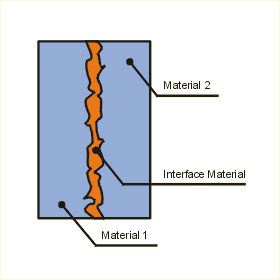Close

This page calculates the temperature difference and the thermal contact conductance (thermal resistance) for the interface formed by two conforming, rectangular, rough surfaces as a function of contact pressure for the low pressure range (between 0.035 and 0.35 MPa). This is commonly occurs in microelectronic applications. This calculator focuses on bare joints filled with air, helium, as well as interfaces filled with thermal greases of varying thermal conductivities. It can be shown that radiation heat transfer is negligible and does not need to be considered for this analysis.

Also note that the interface resistance calculation is a theoretical best, and that bound line thickness, oxide scale, and failure of the interface to wet the surfaces will significantly increase interface resistance.The temperature difference T1-2 is calculated as:

T1-2 = q / (hj x L x W)

Where q is the heat transfer, hj the thermal joint conductance, L the length, and W the width that the two surfaces have in common. Thermal resistance rj is calculated as:

rj = 1 / (L x W x hj)

The thermal joint conductance is calculated as:

hj = hc + hg

Where hc is the thermal contact conductance and hg is the gap conductance. The gap conductance is calculated as:

hg = kg / (Y + M)

The thermal conductivity of the gap substance is given by kg, the effective gap thickness is represented by Y and the gas parameter by M. If the interstitial material is considered a liquid, the gas parameter is entered as zero, and the calculations proceed. The effective gap thickness and the gas parameter are calculated as:

Y = 1.53 sig (P / Hc)-0.097
M = M0 (T / T0) (Pg,0 / Pg)

Where sig is the effective RMS surface roughness of the contacting asperities, P is the contact pressure, and Hc is the surface microhardness of the softer of the two contacting solids. For the calculation of the gas parameter, M0 is the gas parameter at T0 = 50oC and Pg,0 = 1 atm. The gas temperature T and the gas pressure Pg can be defined.

The effective RMS surface roughness is calculated as:

sig = (sig12 + sig22)1/2

Where sig1 is the RMS surface roughness of surface 1 and sig2 that of surface 2.

The contact conductance hc is given by:

hc = 1.25 ks (m / sig) x (P / Hc)0.95

Where ks is the harmonic mean thermal conductivity of the interface, m is the effective mean asperity slope of the interface, sig is the effective RMS surface roughness, P the contact pressure, and Hc the surface microhardness. The harmonic mean thermal conductivity can calculated as:

ks = 2 k1k2 / (k1 + k2)

Where k1 and k2 represent the conductivities of materials one and two respectively.

The effective mean absolute asperity slope of the interface is given by (The with surface roughnesses must be between 0.216 and 9.6 micrometers):

m = 0.125 (sig)0.406

m1 and m2 are the mean absolute asperity slopes of the materials one and two respectively. The mean absolute asperity slope of a given material can be approximated by the same equation.

Although the calculator is mainly for bare surfaces, it can be applied to surfaces with thermal grease. It can be assumed that the grease behaves like a liquid and fills all gaps (wets the interface surfaces completely) between the asperities, then this model is used by substituting M = 0 and the grease thermal conductivity into the gap conductance relationship. This calculator can not be used when solid interstitial materials (i.e. thermal compounds, elastomers, or adhesive tapes) are used.

References

M. Yovanovich, J. R. Culham and P. Teertstra, "Calculating Interface Resistance", Microelectronics Heat Transfer Laboratory, Department of Mechanical Engineering University of Waterloo

Thanks

Brent Webb, Intel Corporation